#The Real Number Line, Interval Notation and Set NotationIn this section, you will learn about three different ways in which to write down sets of solutions.

#### The Real Number Line.

The most intuitive way is to use the real number line. If we draw a line, designate a point on the line to be zero, and choose a scale, then every point on the line corresponds uniquely to a real number, and vice versa: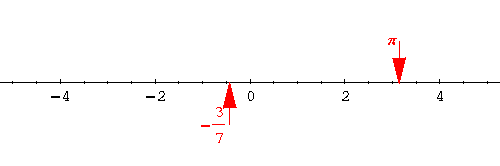The real number line "respects" the order of the real numbers. A bigger number will always be found to the right of a smaller number. In the picture below, a>b.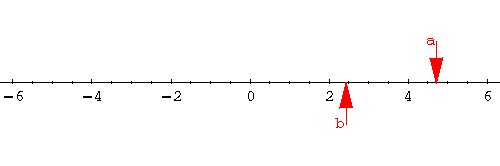We visualize a set on the real number line by marking its members.

It is standard to agree on the following conventions: To include an endpoint, we "bubble it in." To exclude an endpoint, we use an "empty bubble". Here is the set of all real numbers greater than -2 and less than or equal to 5:The number -2 is excluded from the set, so you see an "empty bubble"; the number 5 is included in the set, so the bubble at 5 is "filled in."

Next comes an unbounded set, the set of all numbers less than or equal to 3: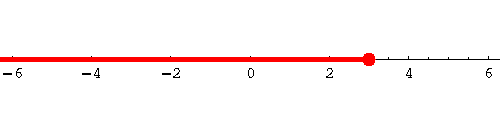The set does not need to be "connected." The following graph depicts all real numbers which are either greater than 2 or strictly between -1 and 1.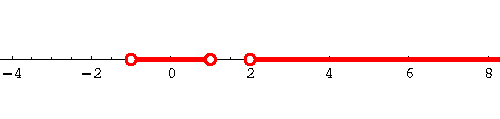The following is a description of the set of all real numbers with the exception of -1 and 2: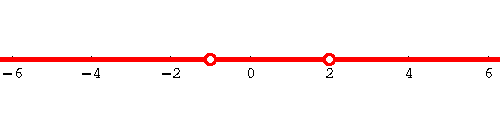#### Interval Notation.

Interval notation translates the information from the real number line into symbols.

Our examplebecomes the interval (-2,5].

To indicate that an endpoint is included, we use a square bracket; to exclude an endpoint, we use parentheses.

Our exampleis written in interval notation as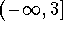. The infinity symbols "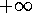" and "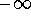" are used to indicate that the set is unbounded in the positive () or negative () direction of the real number line. "" and "" are not real numbers, just symbols. Therefore we always exclude them as endpoints by using parentheses.

If the set consists of several disconnected pieces, we use the symbol for union "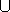":

Our exampleis written in interval notation as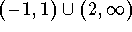.

How could we write downin interval notation? There are three pieces to consider: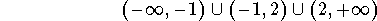An interval such as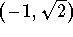, where both endpoints are excluded is called an open interval. An interval is called closed, if it contains its endpoints, such as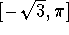.

An unbounded interval such as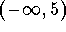is considered to be open; an interval such as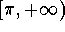is called closed (even though it does not contain its right endpoint). The whole real line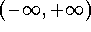is considered to be both open and closed. (So intervals are not like doors, they can be open and closed at the same time.)

#### Set Notation.

The most flexible (and complicated?) way to write down sets is to use set notation.

Sets are delimited by curly braces. You can write down finite sets as lists.

For instance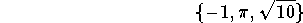is the set with the three elements -1,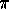and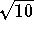.

For sets with infinitely many elements this becomes impossible, so there are other ways to write them down.

Special symbols are used to denote important sets:

• N is the set of natural numbers 1, 2, 3, ...
• Z denotes the integers 0, 1, -1, 2, -2, .... Why Z? The German word for 'number' is 'Zahl'.
• Q denotes the set of rational numbers (fractions). Why Q? I know: the letter R is already taken; Q stands for 'quotient'.
• R denotes the set of all real numbers, consisting of all rational numbers and irrational numbers such as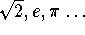.
• C denotes the set of all complex numbers.
•is the empty set, the set which has no elements.

Beyond that, set notation uses descriptions: the interval (-3,5] is written in set notation as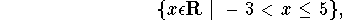read as " the set of all real numbers x such that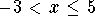."

The first part tells us what "universe" of numbers we are considering (in our case the universe of real numbers), the delimiter "" separates the "universe" part from the second part, where we describe the property our numbers in the set are supposed to satisfy.

The set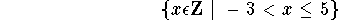is the set of all integers exceeding -3 and not greater than 5; this is a finite set; we could write it as a list,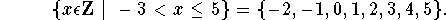The set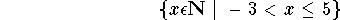is even smaller; it contains only five elements: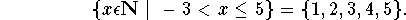Here are some more examples:

The interval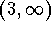can be written as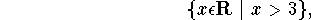the set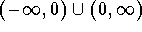looks like this in set notation: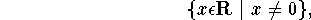or like this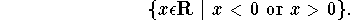#### Exercise 1.

Write the set of all real numbers strictly between -2 andin interval notation and in set notation.

#### Exercise 2.

Write the set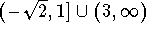in set notation.

#### Exercise 3.

Write the unbounded set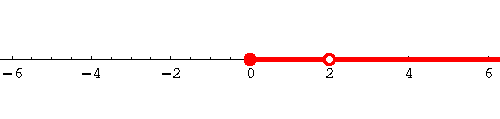in both interval notation and set notation.

#### Exercise 4.

Mark the set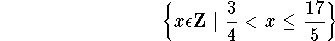on the real number line.

#### Exercise 5.

Write down the set of solutions to the inequality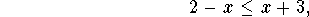in all three notations.[Back] [Next]
[Algebra] [Trigonometry] [Complex Variables]
[Calculus] [Differential Equations] [Matrix Algebra]S.O.S MATHematics home page

Do you need more help? Please post your question on our S.O.S. Mathematics CyberBoard.Helmut Knaust